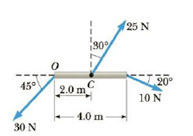Chapter 8, Problem 5P

Chapter
Section
Textbook Problem

Figure P8.4Calculate the net torque (magnitude and direction) on the beam in Figure P8.5 about (a) an axis through O perpendicular to the page and (b) an axis through C perpendicular to the page.Figure P8.5

(a)

To determine

The net torque on the beam about an axis through O perpendicular to the page.

Explanation

Given info: The length of the beam is 4.0m, the distance between points O and C is 2.0m, the forces on the beam are 25N, 10N, and 30N with respect to 30°, 20°, and 30N as shown in the figure.

The following figure shows the forces on the beam.

For net torque on the beam about an axis O, the perpendicular components of forces on the beam are multiplied with their corresponding locations.

The formula for the net torque on the beam is,

τ=aF1cosθ1bF2sinθ2

• a is the distance between points O and C.
• b is length of the beam.
• F1,F2 are forces on the beam.
• θ1 and θ2 are angles of forces.

Substitute 25N for F1, 10N for F2, 30° for θ1, 20° for θ2, 2

(b)

To determine

The net torque on the beam about an axis through C perpendicular to the page.

Still sussing out bartleby?

Check out a sample textbook solution.

See a sample solution

The Solution to Your Study Problems

Bartleby provides explanations to thousands of textbook problems written by our experts, many with advanced degrees!

Get Started

14-12 Write the 1UPAC name of each compound. (e) (f)

Introduction to General, Organic and Biochemistry

What is the nucleolus, and what is its function?

Biology: The Dynamic Science (MindTap Course List)

Review. Find every angle between 0 and 360 for which the ratio of sin to cos is 3.00.

Physics for Scientists and Engineers, Technology Update (No access codes included)